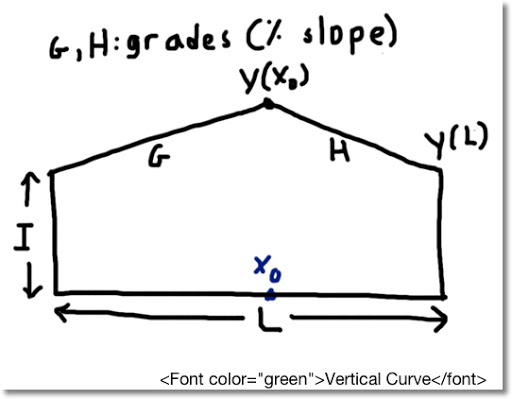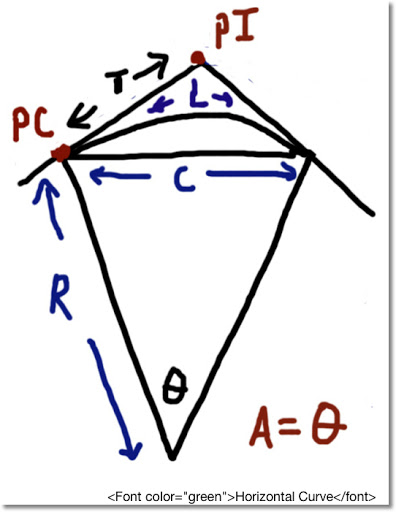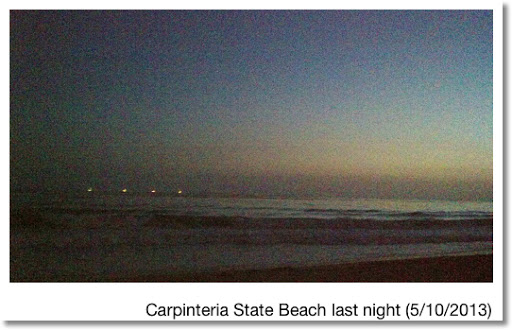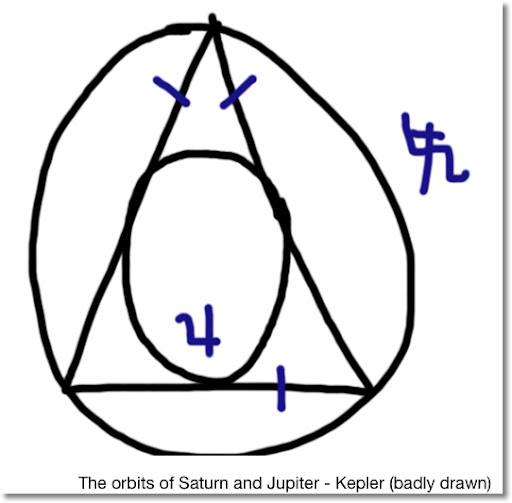## Sunday, May 19, 2013

### HP 35S: Approximate Length of Sunlight During a Day

HP 35S: Length of Sunlight During a Day

Source: Total Daily Amount of Solar Radiation - HP 67/97 Energy Conservation Pac, December 1978, Author: Hewlett Packard

(This is a slight variation instead of a direct port)

Input

You are prompted for D and L where:
D = the number of days from March 21, a 365 day year is assumed
L = latitude (North as positive, South as negative), entered as D.MMSS (degrees-minutes-seconds) format

Output

Approximate number of hours of sunlight, in hours, minutes, seconds

Examples

Los Angeles, April 17: latitude of 34°03' N, 27 days after March 21
D = 27, L = 34.03, answer is approximately 12.573501 (12 hours, 57 minutes, 35.01 seconds)

Rome, September 1: latitude 41°51' N, 164 days after March 21
D = 164, L = 41.52, answer is approximately 12.532269 (12 hours, 53 minutes, 22.69 seconds)

Sydney, June 21: latitude 33°51'31" S, 92 days after March 21
D = 92, L = -33.5131, answer is approximately 9.443922 (9 hours, 44 minutes, 39.22 seconds)

Formulas

This version uses the estimate of sun declination:
D = 23.45 sin(d * 0.9856°)

Since 360/365.25 ≈ 0.985626283368

θ = acos(-tan L × tan D)

L = 24 * θ in radians ÷ π

Program
S001 LBL S
S002 DEG
S003 INPUT D
S004 0.9856
S005 ×
S006 SIN
S007 23.45
S008 ×
S009 INPUT L
S010 HMS→
S011 TAN
S012 x<>y
S013 TAN
S014 ×
S015 +/-
S016 ACOS
S018 24
S019 ×
S020 π
S021 ÷
S022 ->HMS
S023 RTN

This blog is property of Edward Shore. 2013

### HP 35S: Air Density & Density Altitude (Metric-US Conversion Factors included)

HP 35S: Air Density & Density Altitude (7 Digit Accuracy*)

*You can use 4 or 5 digits for the HP 15C and other RPN calculators on which each number takes a step. Slightly less accuracy but smaller program.

Source: Shelquist Engineering web page: http://wahiduddin.net/calc/density_altitude.htm

Formulas

Air Density:

D = P ÷ (R * T)
D = density in kg/m^3
P = pressure in Pascals (Pa)
T = temperature in Kelvins
R = specific gas constant = 287.05 J/(kg*°K)

Air Density Altitude: (where the plane/vehicle thinks it is)
H = 44.3307692 - 42.2665143 * D^0.2349695

H = air density in km

U.S.-SI Conversion Factors
Pressure: (convert and store in P)
1 inHg = 2275.5477799 lb/(ft*s^2) = 3386.3881579 Pa

Temperature Conversion Formula: (convert and store in T)
°K = 5/9 * (°F - 32) + 273.15

Length:
1 km = 3280.8398950 ft

Program
D001 LBL D
D002 INPUT P
D003 287.05
D004 INPUT T
D005 *
D006 ÷
D007 STO D
D008 VIEW D \\ display air density in kg/m^3
D009 0.2349696 \\ dimensionless constant
D010 y^x
D011 42.2665143
D012 *
D013 +/-
D014 44.3307692
D015 +
D016 STO H
D017 RTN \\ display density altitude

I chose to use SI units because the constants are much smaller than the formula would be in US units.

This blog is property of Edward Shore. 2013

### HP35S: Distance to Horizon

HP35S Distance to Horizon

Calculator: HP 35S

Sources:
* HP 65 Navigation Pac-1 - published in 1974
* Wikihow Article: http://m.wikihow.com/Calculate-the-Distance-to-the-Horizon

Input
Height above sea level, the height includes the height of the land (if any) and the distance to your eyes or eyepiece.

Output
Approximate distance to the horizon in miles.

Conversions
If you use metric, use these conversions before/after running the program:
1 m = 3.28083989501 ft
1 mi = 1.609344 km

Formula
Updated formula based on radius on Earth on average 3,959 miles.
D ≈ √(1.49922*H + H^2)
H is in feet, D is in miles

Example
H = 3 ft, D ≈ 3.67392 miles

Program
H001 LBL H
H002 ENTER
H003 ENTER
H004 1.49922
H005 *
H006 x<>y
H007 x^2
H008 +
H009 √
H010 RTN

This blog is property of Edward Shore. 2013

### HP35S Vertical Curve: Elevation at Peak and at End PointHP35S Vertical Curve: Elevation at Peak and Length

Source: Fundamental of Engineering Supplied-Reference Handbook 8th Ed, 2nd Revision, 2011, NCEES

Calculator: HP 35S

Input
I = initial height
G = grade 1; entering the curve (in decimal form)
H = grade 2; exiting the curve (in decimal form)
L = horizontal length of the curve

G and H are the opposite signs

If G>0 and H<0 a="" and="" curve="" g="" h="" has="" if="" peak.="" similarity="" the="">0, the curve has a valley.

The program listed prompts for the inputs.

Output
1. Point where the curve reaches extreme elevation
2. Press R/S to get the elevation at the extreme point.
3. Press R/S once more to get the elevation at the end of the curve.

None of the outputs are stored.

Formula
A = (G - H)/(2L)
X_extrema = -G/(2A)
Equation of the Curve: y = I + G*x + A*x^2

Examples
Uphill curve:
I = 1,000 ft
G = 7% = 0.07
H = -4% = -0.04
L = 1,368 ft

Point at peak elevation is 870.545 ft into the curve at 1,030.469 ft. The elevation at the end of the curve is 1,020.520 ft.

Downhill curve:
I = 1,580 ft
G = -3% = -0.03
H = 4.2% = 0.042
L = 2.3 mi = 12,144 ft

Point at trough elevation occurs 5,060 ft into the curve at elevation 1,504.1 ft. The elevation at the end of the curve is at 1,652.864 ft.

Program
V001 LBL V
V002 INPUT I
V003 INPUT G
V004 INPUT H
V005 INPUT L
V006 RCL H
V007 RCL - G
V008 2
V009 ÷
V010 RCL ÷ L
V011 STO A
V012 RCL G
V013 x<>y
V014 ÷
V015 2
V016 ÷
V017 +/-
V018 R/S \\ shows point where extreme elevation occurs
V019 XEQ V024
V020 R/S \\ shows extreme elevation
V021 RCL L
V022 XEQ V024 \\ shows ending elevation
V023 RTN
V024 ENTER \\ calculate y subroutine
V025 RCL × A
V026 RCL + G
V027 ×
V028 RCL + I
V029 RTN

This blog is property of Edward Shore. 2013

### HP35S: Horizontal Curve - Finding Radius, Chord Length, and Arc LengthHP 35S: Horizontal Curve
Original: HP 33S Surveying Applications, Hewlett Packard, March 1978, pg. 46

Calculator
HP 35S

Input
(see diagram above)

T = Tangent Distance (length of segment from P.C. (Point of Curvature) to P.I. (Point of Tangent Intersection))
A = Central curve in degrees, minutes, seconds

This program prompts for tangent length and central angle

Output

The program gives the following results:
1. Radius of the horizontal curve (R)
2. Press R/S to get the Chord length (C)
3. Press R/S once more to get the arc length of the horizontal curve (L)

The program does not store any results.

Formulas
R = T × (tan(A/2))⁻¹
C = 2 × R × sin(A/2)
L = R × A in radians

Where
T = tangent distance
A = central angle
C = Chord Length
L = Arc Length

Example
Tangent Length: 172.45
Central Angle: 40°22'13" (enter as 40.2213)

Results:
Chord Length: 323.7172
Arc Length: 330.51163

Program
V001 LBL V
V002 DEG
V003 INPUT T
V004 INPUT A
V005 HMS→\\ sometimes named ->H
V006 STO A
V007 2
V008 ÷
V009 TAN
V010 1/x
V011 ×
V013 ENTER
V014 ENTER
V015 2
V016 ×
V017 RCL A
V018 2
V019 ÷
V020 SIN
V021 ×
V022 R/S \\ display Chord Length
V023 x<>y
V024 RCL A
V026 ×
V027 RTN \\ display Arc Length

If you don't have the ->RAD function, you can substitute the following steps:
π, ×, 180, ÷

This blog is property of Edward Shore. 2013

### HP15C: Julian Date from Gregorian Date

Thanks to the University of Texas at San Antonio Computer Science Department. The explanation and formulas can be found by clicking on this link.

Note: This program only works for dates on or after October 15, 1582. Thank you Dieter.

Calculator
HP 15C (can be adopted with any RPN keystroke calculator)

Input
Preload the following information into these registers:
R1 = month (1 for January, 12 for December)
R2 = date
R3 = year in four digits (example: 2013)

Output
R0 = Julian Date

Temporary
R4 = a = integer((14-month)/12)
R5 = y = year + 4800 - a

Formulas
a = integer((14-month)/12)
y = year + 4800 - a
m = month + 12a - 3
Julian Date = day + integer((153m+2)/5) + 365y + integer(y/4) - integer(y/100) + integer(y/400) - 32045

Assumptions: 12:00 PM Universal Time is assumed (that's 7:00 AM in Pacific Standard Time or 8:00 AM in Pacific Daylight Savings Time)

Program
LBL A
1
4
RCL - 1
1
2
÷
INT
STO 4
4
8
0
0
RCL + 3
RCL - 4
STO 5
RCL 2
STO 0
1
2
RCL × 4
RCL + 1
3
-
1
5
3
×
2
+
5
÷
INT
STO + 0
3
6
5
RCL × 5
STO + 0
RCL 5
4
÷
INT
STO + 0
RCL 5
1
%
CHS
INT
STO + 0
RCL 5
4
÷
1
%
INT
STO + 0
RCL 0
3
2
0
4
5
-
STO 0
RTN

In HP RPN calculators, a number followed by 1, %, divides said number by 100.

Example:

March 14, 1977 has a Julian Date of 2,443,217
April 28, 2012 has a Julian Date of 2,456,046
December 31, 2015 has a Julian Date of 2,457,388

This blog is property of Edward Shore. 2013

## Saturday, May 11, 2013

### Greetings from Carpinteria/UCSB - AstronomyI am in Carpinteria, CA; soon to be headed back home (vacation goes by soooooooo fast!)

Last night was a sight to see. Unfortunately I did not catch the young moon, but I did manage to see Jupiter, and the constellations Ursa Major, Leo, Gemini, and Virgo. I can thank Google Sky for the immense help. Who knew the stars of Cancer were so faint?

My trip to the UCSB Library (Davidson Library) completed a circle for me. It was there where I got the itch to visit the mathematics section of as many libraries I can get to. I first visited the UCSB library last September.

This visit I concentrated more on astronomy than mathematics. Learning about the structure of the Milky Way has become an interest for me.

Thanks to Tycho Brahe, comets are a major part of astronomy. He also brought all the astronomical almanacs to date around 1600, all without a telescope. Telescopes were first used by Galileo Galilei. Brahe's data was eventually inherited by Johannes Kepler, who was both an astrologer and astronomer.Kepler showed how proportional the orbits of Jupiter (inscribed circle) and Saturn (circumscribed circle) were (well, in general) by drawing two circles around an equilateral triangle. My attempt at drawing is shown above. (Freehand art was never my strength).

Unlike what astronomers believed before, Galileo showed, among other things:
1. Planets are not self-moving.
2. Stars are not close to the Earth, instead they are distant suns.

The Milky Way

Harlow Shapley was the first to locate the Earth's place in the Milky Way Galaxy (around 1919): in the galactic disc two thirds out from the center. Today, astronomers estimate that we are about 24,500 to 27,000 light years away from the center; which could be a good thing. The center of our galaxy is said to be a massive black hole.

During the time of World War II, H.C. van de Hurst suggested trying to detect radio waves emitted by hydrogen atoms. This opened the door to a more detailed map of our celestial sky.

Source: Charles A. Whitney. "The Discovery of our Galaxy" Alfred A. Knoff: New York. 1971

-------

Currently, the center of our Milky Way lies in the constellation Sagittarius (♐). The biggest identifier is a radio source named Sagittarius A*, which can't be seen by the naked eye. It lies near the boarder of Scorpio (Scorpius) and Ophiuchus.

Coordinates of Sagittarius A* (approximately)
RA (α) 17hr 45min 40.04sec (266.416833°)
Dec (δ) -29°00'28.1" (-29.007806°)

I am curious: with the precision of the equinoxes, will Sagittarius A* "move" to Scorpio and/or Ophiuchus in the far future? It stands to reason, since the point (0hr, 0°), the vernal equinox once lied in the constellation Aries 2,000 years ago, now lies in Pisces on its way to Aquarius.

It is also an explanation why the star Polaris in Ursa Minor and the star Vega in Lyra trade the honor of being the North Star approximately every 12,857 years. (Close to 13,000) Source: http://csep10.phys.utk.edu/astr161/lect/time/precession.html

Hipparchus determined that the stars rotated approximately 50 arc seconds (50".3 to 50".4) around the ecliptic pole. Hence, it takes about 25,714 2/7 years for the stars to complete one cycle. (≈ 360°/50".4 = 360°/0.014°)

Until next time, cheers!

Eddie

## Thursday, May 9, 2013

### Vacation! And some goodies!

Greetings from San Luis Obispo. I am on vacation this week having a great time!

So far I visited Morro Bay (what a sight - despite the presence of a power plant),hung out in downtown SLO, and visited Cal Poly (and it's library - I am going to hit the library at UC Santa Barbara tomorrow).

Some math tips I picked up from my visit:

Fuzzy sets are sets that allow degrees of membership. Instead of having a Yes/No decision of whether an object belongs in a set, degrees of acceptance are allowed.

Let A(x) → [0,1] where A(x) is the degree of acceptance.

The basic properties for normal sets work for fuzzy sets.

From the NIST Handbook of Mathematical Functions (that book is huge!):

Ways to calculate some functions:

Error Function:

erf(z) = 2/√π * ∫(e^(-t^2) dt, 0, z) = 2/√π * Σ((-1)^n * z^(2*n+1) /(n! * (2*n+1)), n=0 to infinity)

Γ(z) = ∫(e^-t * t^(z-1) dt,0,infinity) = (z-1)! = π /(sin (π*z) * Γ(1-z))

Γ(x) ≈ e^-x * x^x * √(2 π/x) * Σ(g_k/x^k, k=0 to infinity) where g_k is from a series. The first few terms are

g_1 = 1
g_2 = 1/12
g_3 = 1/288
g_4 = -139/51840
g_5 = -571/2488320
g_6 = 163879/2090188880

zeta(s) = Σ(n^-s, n=0 to infinity) = 2^(s-1)/Γ(s+1) * ∫(x^s/(sinh x)^2 dx, 0, infinity)
= 1/Γ(s) * ∫(x^(s-1)/(e^x - 1) dx, 0, infinity)

Wishing the best for everyone,

Eddie### TI-Nspire: Templates to Plot Functions, Parametric Equations, and Sequences

TI-Nspire:  Templates to Plot Functions, Parametric Equations, and Sequences Introduction The following are sample templates to plot functi...